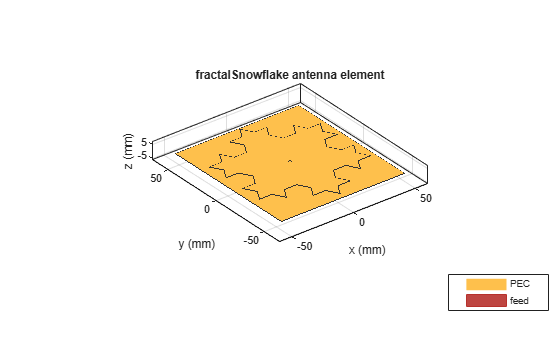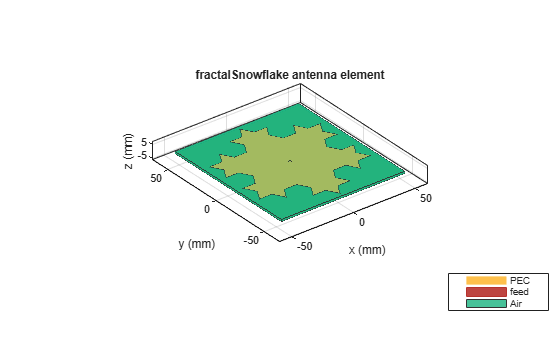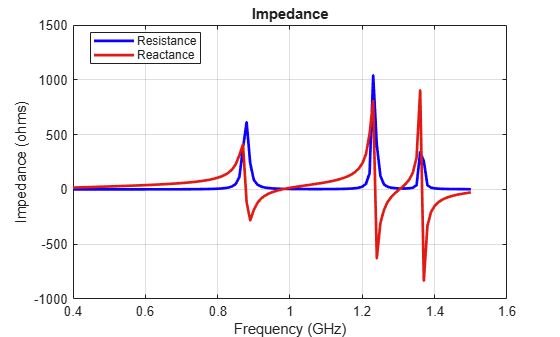# fractalSnowflake

Create fractal Koch snowflake antenna

## Description

The `fractalSnowflake` object creates a Koch snowflake fractal antenna. These fractal antennas are used in mobile phone, Wi-Fi®, and radar applications.A fractal antenna uses a fractal, a self-similar design that is repeated in different dimensions so as to maximize effective the length or increase the perimeter of the material that transmits or receives electromagnetic radiation. This makes the fractal antennas compact and therefore suitable for use in small and complex circuits. Fractal antennas also have higher input impedance or resistance due to their length or increased perimeter.

All fractal antennas are printed structures that are etched on a dielectric substrate.

## Creation

### Syntax

``ant = fractalSnowflake``
``ant = fractalSnowflake(Name,Value)``

### Description

example

````ant = fractalSnowflake` creates a Koch’s snowflake fractal antenna. The default fractal is centered at the origin, and the number of iterations is set to 2. The length of the fractal is for an operating frequency of 4.15 GHz.```

example

````ant = fractalSnowflake(Name,Value)` sets properties using one or more name-value pairs. For example, `ant = fractalSnowflake('Numiterations',4)` creates a Koch's snowflake with four iterations.```

## Properties

expand all

Number of iterations performed on the fractal antenna, specified as a scalar integer.

Example: `'NumIterations',4`

Example: `ant.NumIterations = 4`

Data Types: `double`

Length of the side of the equilateral triangle in fractal snowflake, specified as a positive scalar integer in meters.

Example: `'Length',0.5000`

Example: `ant.Length = 0.5000`

Data Types: `double`

Height of the fractal from the ground plane along z-axis, specified as a positive scalar integer in meters.

Example: `'Height',0.0050`

Example: `ant.Height = 0.0050`

Data Types: `double`

Type of dielectric material used as a substrate, specified as an `dielectric` object. For more information, see `dielectric`. For more information on dielectric substrate meshing, see Meshing.

Example: `d = dielectric('FR4');` `ant = fractalSnowflake('Substrate',d)`

Example: ```ant= fractalSnowflake('Substrate',dielectric('Name','RO4003C','EpsilonR',3.38,'LossTangent',0.0027,'Thickness',0.508e-3))```

Data Types: `string` | `char`

Length of the ground plane, specified as a positive scalar integer in meters.

Example: `'GroundPlaneLength',0.0550`

Example: `ant.GroundPlaneLength = 0.0550`

Data Types: `double`

Width of the ground plane, specified as a positive scalar integer in meters.

Example: `'GroundPlaneWidth',0.0550`

Example: `ant.GroundPlaneWidth = 0.0550`

Data Types: `double`

Signed distance of fractal snowflake center from origin, specified as a two-element real-valued vector with each element unit in meters. The distance is measured along the length and width of the ground plane.

Example: `'FractalCenterOffset',[0 0.080]`

Example: ```ant.FractalCenterOffset = [0 0.080]```

Data Types: `double`

Signed distance of the feed from the origin, specified as a two-element real-valued vector with each element unit in meters.

Example: `'FeedOffset',[0 0.080]`

Example: `ant.FeedOffset = [0 0.080]`

Data Types: `double`

Diameter of the feed, measured in meters.

Example: `'FeedDiameter',0.001`

Type of the metal used as a conductor, specified as a metal material object. You can choose any metal from the `MetalCatalog` or specify a metal of your choice. For more information, see `metal`. For more information on metal conductor meshing, see Meshing.

Example: ```m = metal('Copper'); 'Conductor',m```

Example: ```m = metal('Copper'); ant.Conductor = m```

Tilt angle of the antenna, specified as a scalar or vector with each element unit in degrees. For more information, see Rotate Antennas and Arrays.

Example: `'Tilt',90`

Example: `ant.Tilt = 90`

Example: `'Tilt',[90 90]`,`'TiltAxis',[0 1 0;0 1 1]` tilts the antenna at 90 degrees about the two axes defined by the vectors.

Note

The `wireStack` antenna object only accepts the dot method to change its properties.

Data Types: `double`

Tilt axis of the antenna, specified as:

• Three-element vector of Cartesian coordinates in meters. In this case, each coordinate in the vector starts at the origin and lies along the specified points on the X-, Y-, and Z-axes.

• Two points in space, each specified as three-element vectors of Cartesian coordinates. In this case, the antenna rotates around the line joining the two points in space.

• A string input describing simple rotations around one of the principal axes, 'X', 'Y', or 'Z'.

Example: `'TiltAxis',[0 1 0]`

Example: `'TiltAxis',[0 0 0;0 1 0]`

Example: `ant.TiltAxis = 'Z'`

Note

The `wireStack` antenna object only accepts the dot method to change its properties.

Data Types: `double`

Lumped elements added to the antenna feed, specified as a `lumpedelement` object. You can add a load anywhere on the surface of the antenna. By default, the load is at the feed. `lumpedelement` is the object for the load created using `lumpedElement`. For more information, see `lumpedElement`.

Example: `'Load',lumpedelement`.

Example: ```ant.Load = lumpedElement('Impedance',75,'Frequency',2.9e6,'location',[20e-3 1e-3 1.5e-3])```

## Object Functions

 `show` Display antenna or array structure; display shape as filled patch `impedance` Input impedance of antenna; scan impedance of array `sparameters` Calculate S-parameter for antenna and antenna array objects `rcs` Calculate and plot radar cross section (RCS) of platform, antenna, or array `returnLoss` Return loss of antenna; scan return loss of array `vswr` Voltage standing wave ratio of antenna `optimize` Optimize antenna or array using SADEA optimizer `pattern` Radiation pattern and phase of antenna or array; Embedded pattern of antenna element in array `patternAzimuth` Azimuth pattern of antenna or array `patternElevation` Elevation pattern of antenna or array `axialRatio` Axial ratio of antenna `beamwidth` Beamwidth of antenna `current` Current distribution on metal or dielectric antenna or array surface `charge` Charge distribution on metal or dielectric antenna or array surface `efficiency` Radiation efficiency of antenna `EHfields` Electric and magnetic fields of antennas; Embedded electric and magnetic fields of antenna element in arrays `mesh` Mesh properties of metal or dielectric antenna or array structure `design` Design prototype antenna or arrays for resonance around specified frequency

## Examples

collapse all

Create and View fractal Koch snowflake antenna object with default properties.

`ant = fractalSnowflake`
```ant = fractalSnowflake with properties: Length: 0.0900 NumIterations: 2 Height: 0.0015 Substrate: [1x1 dielectric] GroundPlaneLength: 0.1000 GroundPlaneWidth: 0.1100 FractalCenterOffset: [0 0] FeedOffset: [0 0] FeedDiameter: 0.0020 Conductor: [1x1 metal] Tilt: 0 TiltAxis: [1 0 0] Load: [1x1 lumpedElement] ```
`show(ant)`Create and view fractal Koch snowflake on a substrate with a dielectric constant of 4 and thickness of 1.5e-3.

``` ant = fractalSnowflake('Substrate', dielectric('EpsilonR',4,... 'Thickness',1.5e-3)); show(ant);```Create a fractal Koch snowflake antenna and plot its impedance over a frequency range of 400-1500 MHz.

```ant = fractalSnowflake('Length',180e-3,'GroundPlaneLength',280e-3,... 'GroundPlaneWidth',240e-3,'Height',5e-3,'FeedOffset',... [75e-3,-45e-3]); figure impedance(ant,(400:10:1500)*1e6)```## Version History

Introduced in R2020a